# TCS NQT Numerical Ability Questions

31 August 2023

TCS NQT Numerical Ability Foundation Section Previous Year Questions

## Get 30 minutes free mentorship by TalentBattle Placement Experts

Verbal Ability (Foundation)

TCS NQT Numerical Ability

(Foundation Section Previous Year Questions)

Q1) A man has to travel 50 km in two hours. He could cover 20 km in one hour and then had to stop for 10 minutes for refuelling. By what factor should he increase his speed with reference to that during the first hour so as to be able to complete the journey as per schedule?

A.  1.2

B.  1.8

C.  2.4

D.  1.5

Explanation: Given that,

Total distance covered by man in 2 hours = 50km.

in first hour, he covers = 20km.

So,

Distance left to be cover = 50 - 20 = 30km.

Time left = 2 - 1 = 1 hour.

now, given that, he had to stop for 10 minutes for refuelling.

So,

Time left for travel = 1 hour - 10 min. = 60 - 10 = 50 minutes.

Therefore,

Distance to be covered now = 30km.

Time left = 50 minutes = (50/60) = (5/6) hours.

New speed = Distance/Time = 30/(5/6) = 30 * (6/5) = 36 km/h.

Hence,

Speed in first hour = 20km/h .

speed in second time = 36km/h.

Increased speed in factor = (36 /20) = 1.8 times. (Ans.)

So, he has to increased his speed 1.8 times in order to complete the journey as per schedule time.

Q2) What is the diameter in cm of a solid right circular cylinder whose height is 6 cm and the area of the curved surface is five times the combined area of the two flat surfaces?

A. 3

B.  2.4

C.  1.2

D.  0.9

Explanation: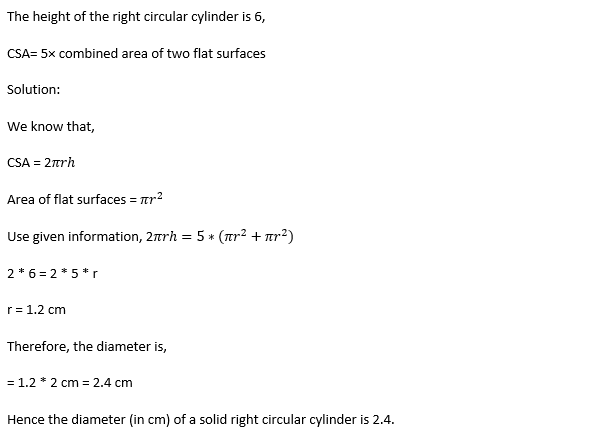Q3) If n is an integer such that 1nn352 is a six-digit number exactly divisible by 24, What will be the sum of the possible values of n?

A. 15

B.  27

C.  9

D.  21

Explanation: we know that,

If the sum of the digits of a number is divisible by 3, then the number is divisible by 3.

A number is divisible by 8 if the last three digits are evenly divisible by 8.

If the number is separately divisible by 3 and 8 then the number is also divisible by 24.

So, we can conclude that, if given 6 digit number is divisible by 24, it must be divisible by 3 and 8.

checking by 8 first we get: -

352/8 = 44 quotient, 0 remainder.

So, it is divisible.

now, in order to divisible by 3, sum must be divisible by 3.

So,

(1 + n + n + 3 + 5 + 2) / 3 = 0 remainder.

(11 + 2n) / 3 = 0 remainder.

Putting values of n now, we get,

if n = 0 => 11 /3 = Remainder not equal to 0.

if n = 1 => (11 + 2)/3 = Remainder not equal to 0.

if n = 2 => (11 + 4)/3 = Remainder equal to 0.

if n = 3 => (11 + 6)/3 = Remainder not equal to 0.

if n = 4 => (11 + 8)/3 = Remainder not equal to 0.

if n = 5 => (11 + 10)/3 = Remainder equal to 0.

if n = 6 => (11 + 12)/3 = Remainder not equal to 0.

if n = 7 => (11 + 14)/3 = Remainder not equal to 0.

if n = 8 => (11 + 16)/3 = Remainder equal to 0.

if n = 9 => (11 + 18)/3 = Remainder not equal to 0.

Therefore,

→ sum of Possible values of n = 2 + 5 + 8 = 15 (Ans.)

Q4) What is mean proportional (MP) between the MPs of (2/7 & 32/343) and (2 & 1/5000)?

A. 3/35

B. 4/35

C. 2/35

D. 2/175

Explanation: Let the MP of 2/7 and 32/343 be x

x = √(2/7)*(32/343)

= √(64/4901)

= (8/49)

Let the MP of 2 and 1/5000 be y

y = √(2)*(1/5000) = √1/2500 = 1/50

MP of x and y = √(8/49)*(1/50) = √8/2450

= √4/1225 = 2/35

Q5) How much percentage is (0.025% of 240% of 1.5) of 0.9?

A. 0.01

B. 10

C. 0.1

D. 1

Explanation: (0.025/100) * (240/100) * 1.5

= 1/4000 * 24/10 * 1.5

= 9/10000

Let x% of 0.9 be 9/10000

x% (0.9) = 9/10000

(x/100) (0.9) = 9/10000

x = (9/10000)*(100/0.9)

x = 0.1

Q6) The cost of filling a gas tank at a shop is Rs. 800. If the shopkeeper reduces the price by 15%.The number of his customers increases by 30%. By what % did his revenue decrease/increase.?

A. 10.5%

B. 10%

C. 8%

D. 12.5%

Explanation: Let initial number of customer's are x .

So,

Initial cost of filling a gas tank = Rs.800

Initial number of customer's = x

then, Initial total revenue = 800 * x = Rs. 800x

Now,

New cost of filling a gas tank = 15% reduced = (85 * 800)/100 = Rs. 680

New number of customer's = 30% increases = (130 * x)/100 = 1.3x

then, New total revenue = 680 * 1.3x = Rs. 884x

therefore,

Revenue increased by = 884 - 800 = Rs. 84x

hence, Revenue increased in % = (84x * 100)/800x = 10.5%

Q7) Rahl takes a sum of Rs. 2310 as a loan. He has to repay this in two equal annual installments. If the rate of interest is 20% compounded annually, what percent of the principal amount taken by  Rahul is the total interest paid by him.

A. 20%

B. 40.9%

C. 130.9%

D. 44%

Explanation: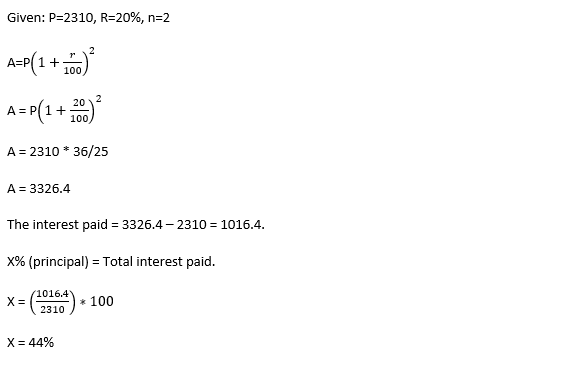Q8) Two numbers are in the ratio of 3:5 . If 3 is added to the first number and 9 is added to the 2nd number, their ratio becomes 4:7. The sum of the original numbers is.

A. 120

B. 150

C. 105

D. 135

Explanation: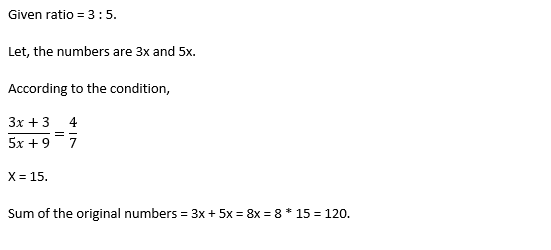Q9) Ankush bought (x+2) apples at the rate of Rs. 12 each. One apple got rotten during transportation and he sold all the remaining apples for Rs. 300. If the percentage profit made by  Ankush in the whole transaction is 25%, find the number of apples sold by Ankush.

A. 19

B. 15

C. 10

D. 12

Explanation: Here it is given that Ankush bought (x+2) apples at the rate of Rs. 12 each.

So total cost price = Rs 12(x + 2)

Now one apple got rotten during transportation

So, number of apples sold = x + 2 - 1 = x + 1

Total selling price = Rs 300

Profit percentage = 25%

So, by the given condition,

12(x+2) * 125/100 = 300.

X = 18

Hence the number of apples sold by Ankush

= 18 + 1

= 19

Q10) An article is marked 36% above its cost price. A discount of 10% is offered on the marked price. Later on, the article was sold by giving another discount of Rs.12.60. If there is a profit of 15.4%, the marked price (in Rs.) of the article is:

A. 245

B. 243

C. 220.30

D. 244.80

Explanation: Let CP of article = Rs. 100x

MP = 36% above CP = (136 * 100x)/100 = Rs. 136x

Discount = 10%

SP = MP * (100 - D)/100 = (136x * 90)/100 = Rs. 122.4x

now,

Another discount = Rs.12.60

SP after another discount = Rs. (122.4x - 12.6)

According to the question,

Total Profit = 15.4%

122.4x - 12.6 = (100x * 115.4)/100

122.4x - 12.6 = 115.4x

7x = 12.6

x = 1.8

therefore,

MP of article = 136x = 136 * 1.8 = Rs. 244.80

Q11) The ratio of 25% of x to 65% of y to 70% of z is 5:26:15. If one sixteenth of their sum(x,y and z) is equal to 10. What is the value of X-2Y+3z?

A. -20

B. -10

C. 10

D. 0

Explanation: 25% of x: 65% of y : 70% of z = 5 : 26 : 15

(25 * x)/100 : (65 * y)/100 : (70 * z)/100 = 5 : 26 : 15

5x:13y:15z = 5:26:15

5X = 5a

x = a

and 13Y = 26a

Y = 2a

and, 15Z = 15a

Z = a

also,

(1/16) (x + y + z) = 10

(x + y + z) = 160

a+2a+a = 160

a=40

therefore, x = 40, Y = 80 and Z = 40

(x - 2y + 3z) = 40 -160 +120 = 0

Q12) A boat travels 12 km downstream and 6 km upstream in 3 hours. The same boat takes fifty percent extra time to cover 10 km  downstream and 16 km upstream. If the same boat travels 20 km downstream and z km upstream in 4 hours find z.

A. 9km

B. 7.5km

C. 8km

D. 4.5km

Explanation: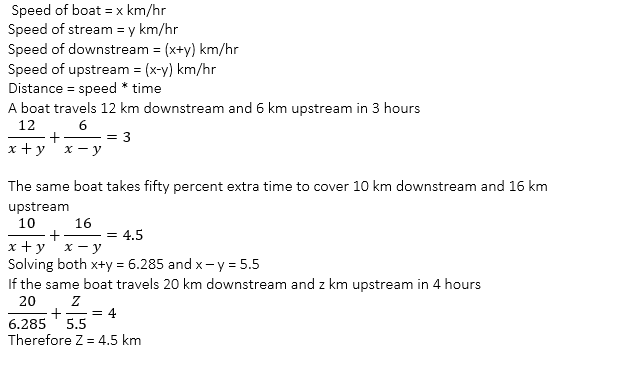Q13)  The savings of surekha are equal to 40% of her expenditure. If her income increases by 20% and the expenditure increases by 40%, her savings decreases by:

A. 20%

B. 30%

C. 50%

D. 10%

Explanation: Let saving = 40, expenditure = 100 hence income = 140

Income increases by 20% = 168, expenditure increases by 40% = 140, saving = 28

% decrease = 40-28 /40 * 100 = 30 %

Q14) The shopkeeper sold some chairs for Rs. 3240 and made a profit of 20%.What was the profit percentage if he had sold chairs for Rs. 2781????????

A. 4.25%

B. 3%

C. 4.5%

D. 3.25%

Explanation: Profit = 20%

Hence 120% of cost price = 3240

Cost price = 2700

New selling price = 2781/- , profit = 81

% profit = 81 / 2700 * 100 = 3%

Q15) If 20% apple from a cart are rotten and the remaining apple are sold at 20% discount the seller gets 92%profit on the cost price of the whole cart by what percent above the cost price
Has the seller marked up the apples?

A. 180%

B. 250%

C. 280%

D. 200%

Explanation: Let there be 100 apples with a cost price of 100 and cost amount=100*100=10000

Sale amount at 92% profit over all=10000*192/100=19200

Number of rotten apples at 20%=20/100*100=20

Number of saleable apples =100-20=80

Sale price at 92% profit over all =19200/80=240

Marked price to allow discount at 20%=240/80*100=300

Mark up=mp-cp=300–100=200

Percentage of mark-up=200/100*100=200%

Q16) Out of 80 students 45%, students passed in Mathematics and 60% students passed in English. If 35% students failed in both the subjects, how many students passed in both the subjects?

A. 24

B. 28

C. 32

D. 20

Explanation: 45% of 80 = 36

60% of 80 = 48

Total passed = 80 - 35% of 80 = 80 - 28 = 52

passed in both = 48+36-52= 32

Q17) 50 workers can construct a bridge in 40 days by working 8 hrs per day. But they completed 30 days and completed only half of the work. How many more workers are needed to complete the work on time by working 10 hrs per day?

A. 65

B. 50

C. 60

D. 70

Explanation: Total Days = 40

Days gone = 30

Days left = 40 - 30 = 10

number of hours per day = 10 hrs

as work is same

M1*H1*D1 = M2*H2*D2

50*8*30 = (50+X) * 10 * 10

X = 70

Q18) The ratio of the speed of a bus and a car is 2:5. The car covers a distance of 280 km in some time and  the bus covers a distance of “d” km less in the same time. What is the distance covered by the bus?

A. 112 km

B. 144 km

C. 168 km

D. 132 km

Explanation: Speed of bus = 2x km/hr

Speed of car = 5x km/hr

Distance = Speed * time

280 = 5x * T

distance covered by the bus = y

y = 2x * T

280/y = 5/2

y = 280 * 2/ 5

y = 112

distance covered by the bus = 112  km

Q19) The sum of the present ages of Ajay and his wife Sushma is 82 years. Sushma’s present age is 28 years more than Sashank, her son. If Sashank’s present age is 12 years, what will be the sum of ages of Sashank and his father after 4 years?

A. 56 years

B. 60 yeras

C. 62 years

D. 58 Years

Explanation: Age of Ajay  + Sushma = 82

Shashank = 12 , hence Sushma = 28+12 = 40

Ajay = 82-40 = 42

Sashank after 4 years = 16

Ajay after 4 years = 46

Sum = 16+46 = 62 years

Q1) A sum of Rs 7500 amounts to Rs 9075 at 10% p.a in a certain time, when the interest is compounding annually.

What is the amount in (Rs) of the sum at the same sum at the same rate for 6/5th of the earlier time.

A. 9412

B. 9427

C. 9680

D. 9580

Explanation: From condition 1,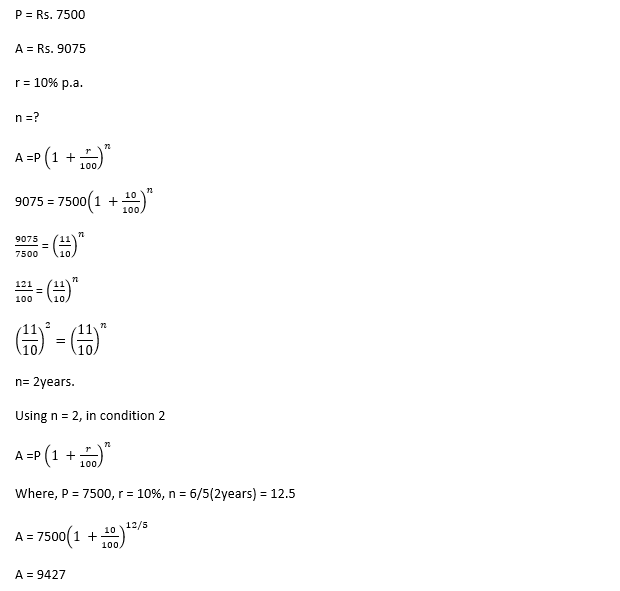Q2) The average weight of some students in class is 62kg. If 8 students of average weight 55kg leave the class and13 students of average weight 65 kg joins the class, then the average weight of remaining students in the class is 63.9 kg. The number of students in the class initially was?

A. 45

B. 55

C. 40

D. 50

Explanation: We can solve this question easily using linear equations and the concept of average.

Let the number of students initially present in the class be = x

then the weight of all the students = 62x (? total = avg × no. of things)

when 8 students leave the class, the weight of the remaining

students = 62n - 8(55)

and when other 13 students join the class, the weight of all students =

62n - 8(55) + 13(65) = 63.9 (n - 8 + 13)

62n = 63.9(n+5) + 440 - 845

62n - 63.9n = 319.5 - 405

- 1.9n = -85.5

x = 45

∴ The total number of students in the class initially were 45.

Q3) Ramesh can complete a work in 20 days. Mohan is 66.67% as efficient as Ramesh. Mohan and Ramesh work together. Ramesh leaves after working for some days. The remaining work is done by Mohan in 10 days. After how many days did Ramesh leave the work?

A. 10 days

B. 6.5 days

C. 8.5 days

D. 8 days

Explanation: Given,

Ramesh completes work in 20 days

Let the Total work to be completed = 1 (1 unit)

So,

Ramesh can complete (1/20) part of work in 1 day

Given,

Mohan is 66.67% as efficient as Ramesh

i.e., If Ramesh completes X part of work in 1 day, then Mohan completes 2X/3 part of work in 1 day.

Here, Ramesh completes (1/20) part of work in 1 day

So, Mohan can complete (2/3)*(1/20) = (1/30) part of work in 1 day

Mohan can complete work in 30days

Let D be the days Ramesh has worked i.e., after D days Ramesh left the work.

Both Ramesh and Mohan can complete (1/20+1/30) part of work in 1 day.

In D days Ramesh and Mohan can complete

D*(1/20+1/30) part of work

In 10 days Mohan can complete

10*(1/30) part of work

We have,

D*(1/20+1/30) + 10*(1/30) = 1

L.C.M of (20,30) = 60

D*(3+2) + 10*2 = 60

5D + 20 = 60

5D = 40

D=8

Therefore, after 8 days Ramesh left the work

Q4) When a number x is divided by 9, the remainder is 6. When the same number is divided by 21, the remainder is 12. If the x lies between 250 and 400, then what is the sum of all possible values of x.?

A. 1107

B. 855

C. 1044

D. 666

Explanation:

x  =    9A + 6

x =    21B  + 12

9A + 6 = 21B + 12

9A = 21B + 6

3A = 7B  + 2

B = 1, A = 3          => x = 33

B =4, A  = 10     => x =  96

B = 7, A = 17       => x =  159

and so on x = 222, 285, 348, 411

x between 250 and 400

So, x can be 285, 348

Sum = 285 + 348 = 633

sum of all possible values of x is 633.

Q5) A bag contains x+5 yellow balls, 2x+1 blue balls and some red balls. If two balls are drawn one after another from the bag without replacement, then the probability of getting a red and blue ball is 1/6. The total balls in the bag is 4(x+2). Find the number of red balls.

A. 8

B. 4

C. 6

D. 5

Explanation: Yellow balls are = x + 5

Blue balls are = 2x + 1

Red balls =?

Total balls = 4(x + 2) = 4x + 8, So red balls are = x + 2.

Find value of x, to answer how many red balls are there.

Given, two balls were selected and the probability of getting a red and blue is 1/6.

From this,

{(x+2)C1 * (2x+1)C1}/(4x+8)C2 = 1/6

Solving above equation gives x = 2.

So total red balls are = x +2 = 2+2 = 4. Option B

Q6) The taxi charges in a city consist of a fixed charge together with the fixed charge for the distance travelled in kilometers. When a person travels 72 km he pays 1107. He pays Rs. 898 for travelling 55 km. What will he have to pay for travelling 45 km?

A. Rs. 826

B. Rs. 740

C. Rs. 693

D. Rs. 774

Explanation: Let, fixed charged be = Rs. X

And charge per km = Rs. Y

1st journey, X + 72y = 1107

2nd journey, X + 55y = 898.

Solving both Y = Rs. 12.3.

Substituting Y in any one of the equations gives, X = Rs. 221

Then for 3rd journey, X + 45y = 221 + 45*12.3 = 774

Q7) C can complete the work alone in 60 days. A and B take 40% and 75% more time than C. The work was started by A and B, and C worked with A on every third day, In how many day the work will be completed.

A. 16(4/5)

B. 48(1/4)

C. 16(1/4)

D. 48(4/5)

Explanation: C can complete the work alone in 60 days.

A can complete work in 60 + (40/100)60  = 84 days

B can complete work in 60 + (75/100)60  = 105 days

Work Done in 3 Days =

3 * (1/84) + 1/105 + 1/60 = (15 + 4 + 7)/420 =26/420

= 13/210

Work done in 16 * 3 = 48 days

= 16 * 13/210 = 208/210

Work left = 2/210 = 1/105

1/105 Work done by A in (1/105)/(1/84) = 4/5  day

Hence work will be completed in = 48 4/5 Days

Q8) A and B start from the same point and cover equal distances. A travels by car and covers the distance in 3 hours with a speed of 50 km/h. The B travels by bus which stops for 10 mins after covering 10 kms. In how much time will the bus reach the destination if the speed of the bus is 40% less than the speed of the car?

A. 6 h 40 min

B. 7 h

C. 7 h 30 min

D. 7 h 20 min

Explanation: Distance = speed × time

total distance = 50km×3hr= 150km

speed of bus is

50 - 40% of 50 = 30km/hr

time taken by bus without stopping

150/30 =5hr

since bus stops for 10 min at each 10km

150km/10km = 15 stops

but last stop we are not considering

because its the destination.

14 stops ×10 min =140 min

so total time spent will be 5hr + 140min i.e.,

7hr 20min

Q9) A sum when lent at the rate of 15% p.a.simple interest for x years amounted to 17,600.When the same sum was lent at the rate of 18% p.a. The Simple interest for (x+2.5) years, it amounted for 24,320. The value of x and the sum, respectively are?

A. 2.5 and 12500

B. 2.5 and 12800

C. 2 and 12500

D. 3.5 and 12800

Explanation: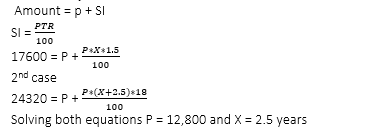Q10) Raj sold his bat at x% profit after giving a discount of x%. The marked price was Rs. 2,400 more than the cost price. And the selling price was Rs. 900 more than the cost price. Find the value of 4x.?

A. 100

B. 50

C. 40

D. 200

Explanation: Selling price = 900 + C.P

Mark price = 2400 + C.p

Discount = M.P – S.P = 1500

Profit = S.P – C.P = 900

X% of C.p = 900

X% of MP = 1500

X% of ( 2400 + CP ) = 1500

X% of 2400 + x% of cp = 1500

X% of 2400 + 900 = 1500

X% of 2400 = 600

X = 25

Value of 4x = 100

Q11) If the average of five consecutive odd number is 27, then what will be the sum of the largest number and the average?

A. 63

B. 62

C. 61

D. 58

Explanation: Let numbers are X, X+2, X+4, X+6, X+8

Average = sum /5

Sum = 5X + 20

5X +20 = 27*5

X = 23

Largest number = X+8 = 23+8 = 31, average = 27 sum = 31+27 = 58

Q12) A Car covers the distance between two points in 45minutes. If the speed of the car is reduced by 5km/hr, the time taken to cover the distance increases to 48minutes. What is the distance between the two points?

A. 55km

B. 50km

C. 60km

D. 45km

Explanation: Let distance = X km

And speed = Y km/hr

A Car covers the distance between two points in 45minutes

X/Y = 45/60 hrs

x/y = ¾

4x = 3y

Y = 4x/3

If the speed of the car is reduced by 5km/hr, the time taken to cover the distance increases to 48minutes

X / (y-5) = 48/60

X / (y-5 ) = 4/5

5x = 4y – 20

Sub Y = 4x/3 value of X = 60km

Q13) A Sum of money, when invested at 20% interest per annum, compounded half-yearly, amounts to a total of Rs.1,331 after a year. What is the sum of money that had been invested????????

A. 1,120

B. 1,110

C. 1,100

D. 1,125

Explanation: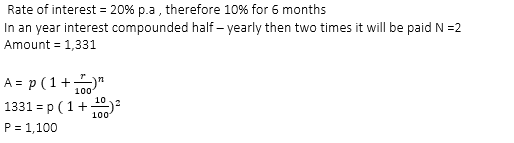Q14) A shopkeeper bought a lamp at rs.1200 and the ratio of its cost price and marked price was 3:5. it was said after two successive discount of 30% and 18% and incurred a loss or profit of x%. Find x.

A. 4  2/3 profit

B. 4  2/3 loss

C. 4  1/3 loss

D. 4  1/3 profit

Explanation: C.P = 1200

C.P : M.P = 3:5

Hence M.P = 2000

After 1st discount of 30% on 2000 = 600 will be reduce

M.P = 1400 2nd discount of 18% of 1400 = 252 will be reduced

S. P = 1148

LOSS = 1200 – 1148 = 52

% loss = 52 / 1200 * 100 = 4(1/3)loss %

##